This small blog post is about calculating and visualising trends based on Covid-19 raw data from the COVID-19 Data Repository by the Center for Systems Science and Engineering (CSSE) at Johns Hopkins University. This is a simple exercise that tries to form a first-hand impression on how Covid-19 cases are trending on a country basis.

### Trend Calculation

We have used the Mann-Kendall Test to determine whether a time series has a monotonic upward or downward trend. This test should work well for all types of distributions and other problems that have no seasonality effects. This type of test does not require that the data be normally distributed or linear and can have four outcomes in terms of trending:

• increasing
• decreasing
• no trend
• failure (not enough data)

The library we used for calculating this trending test was:

https://pypi.org/project/pymannkendall/

### Data

It is not difficult to access the data of the Johns Hopkins University. You just need git. To get the data, you only need one command:

git clone https://github.com/CSSEGISandData/COVID-19.git

The data you get from this repository comes as multiple CSV files and in order to produce a dataset - which you can use for creating diagrams - you will need to concatenate the files and then perform some basic grouping operations on it.

For trending, we have used the Mann-Kendall Test. Still, we also used for drawing lines in the diagrams ridge regression (with the help of the Python package scikit learn) which is a form of linear regression better suited to prevent over-fitting. So this regression type essentially draws a line that based on a parameter can be more or less biased towards the input data.

We have created some simple diagrams for each country over windows of 30 days which depict the trend of active cases, but you could apply the recipe to other features of the dataset. Here is an example for the US showing the trends of active cases over the period of 3 months: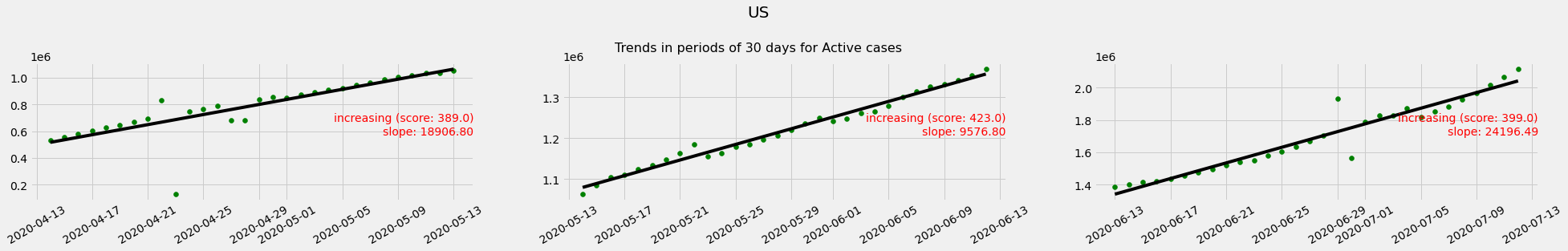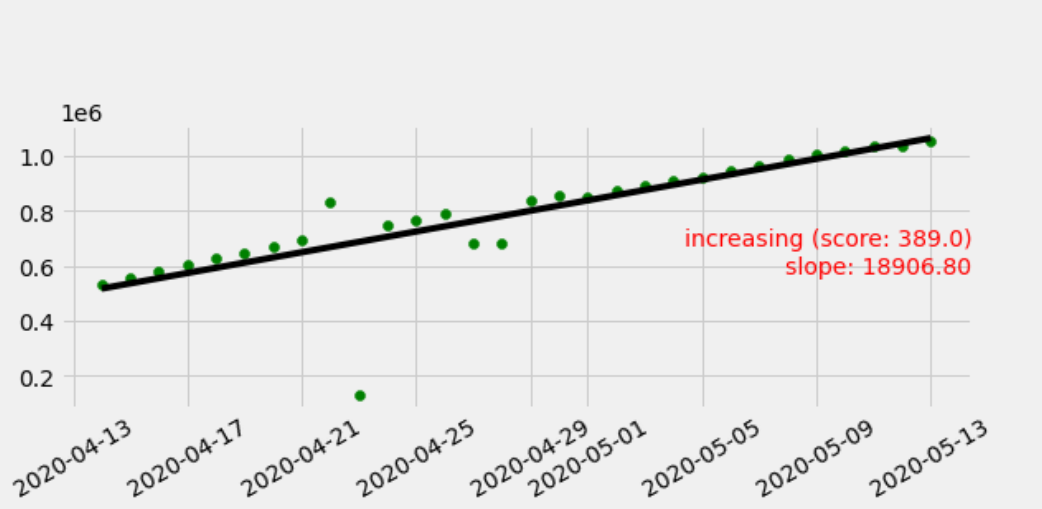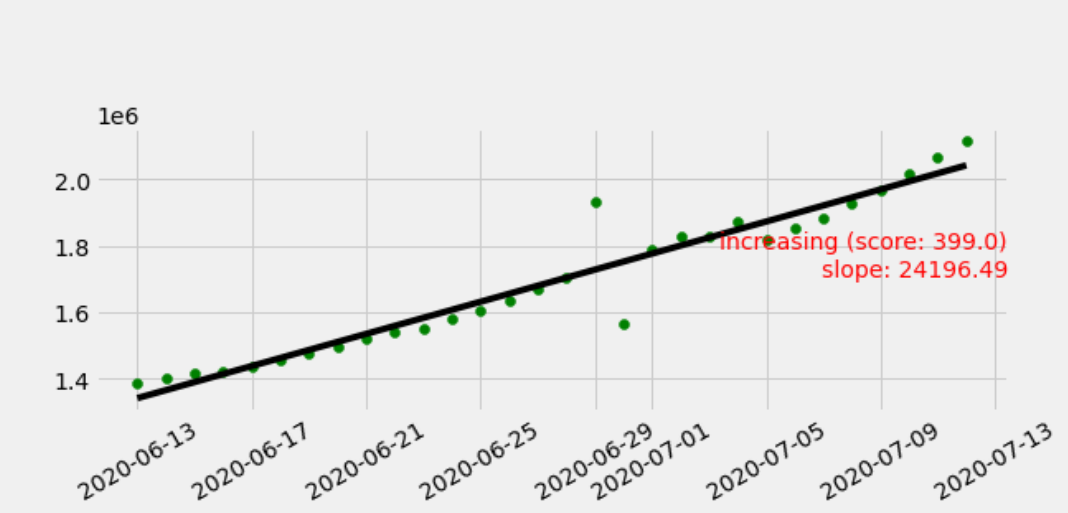The trend is typically associated with the slope given by the ridge regression model. Typically a positive slope indicates an upward trend, and a negative slope means that the tendency is for the active cases to become less. Yet, the actual trend according to the Mann-Kendall Test also includes other variables like the significance level which might influence the verdict of the test, i.e., you can have according to this test a negative slope, but no trend.

In the case of the US, the trend was always uphill, and the slope became steeper in the last 30 days.

The case of Germany, as another example, shows a different evolution. For a while, the trend was going down and then no recognisable trend.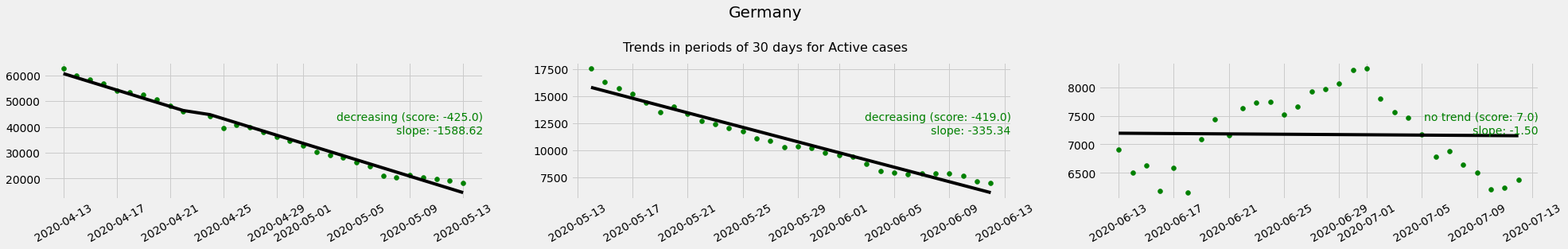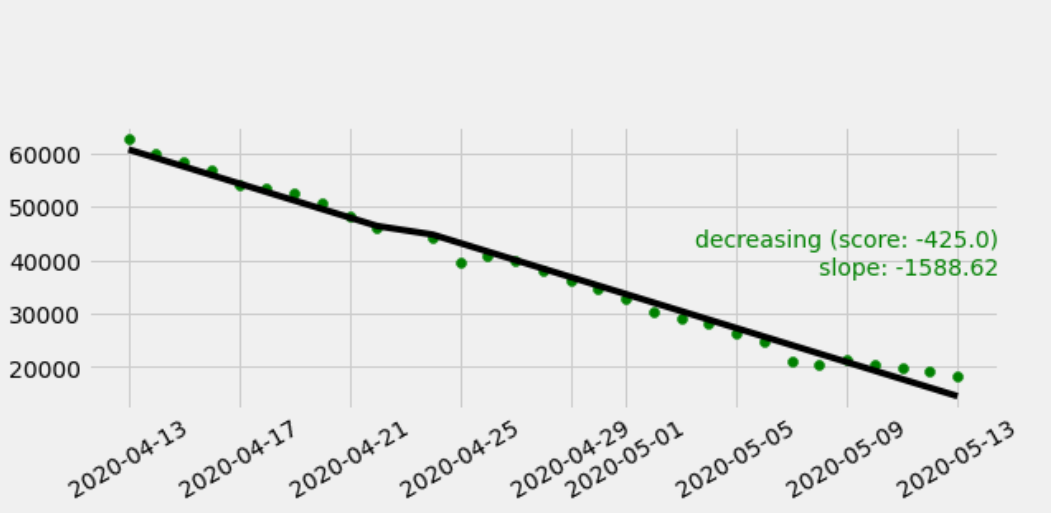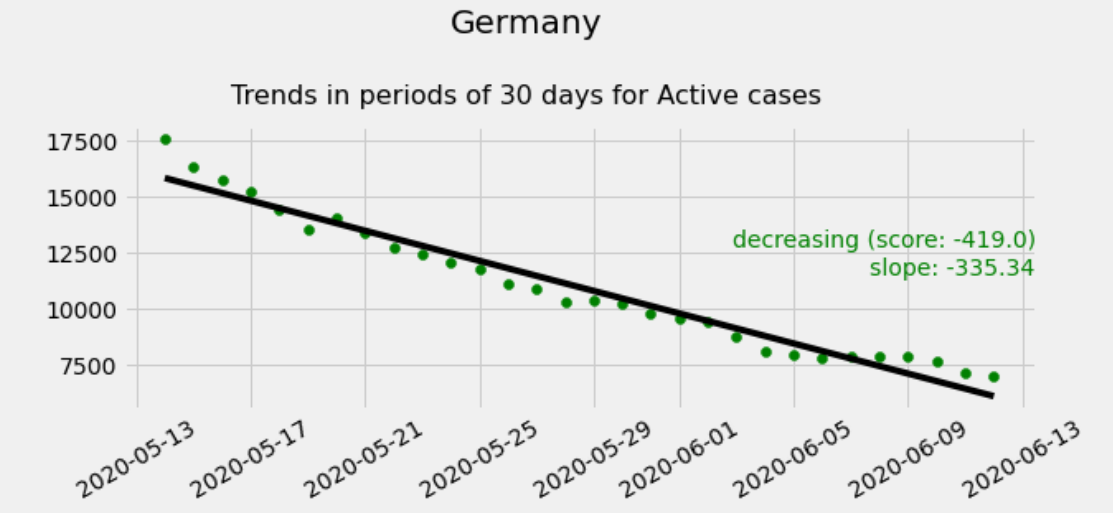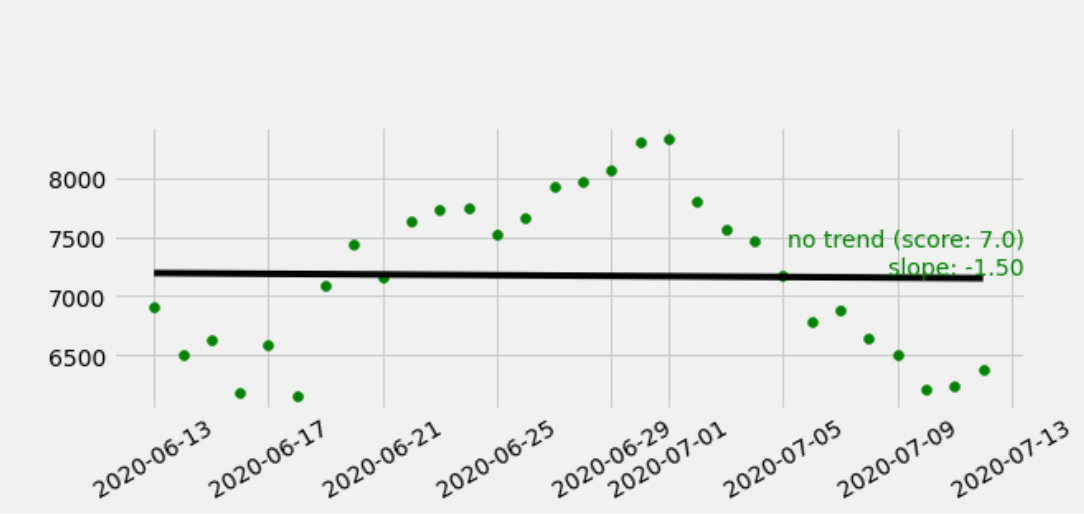Here are the examples for Russia: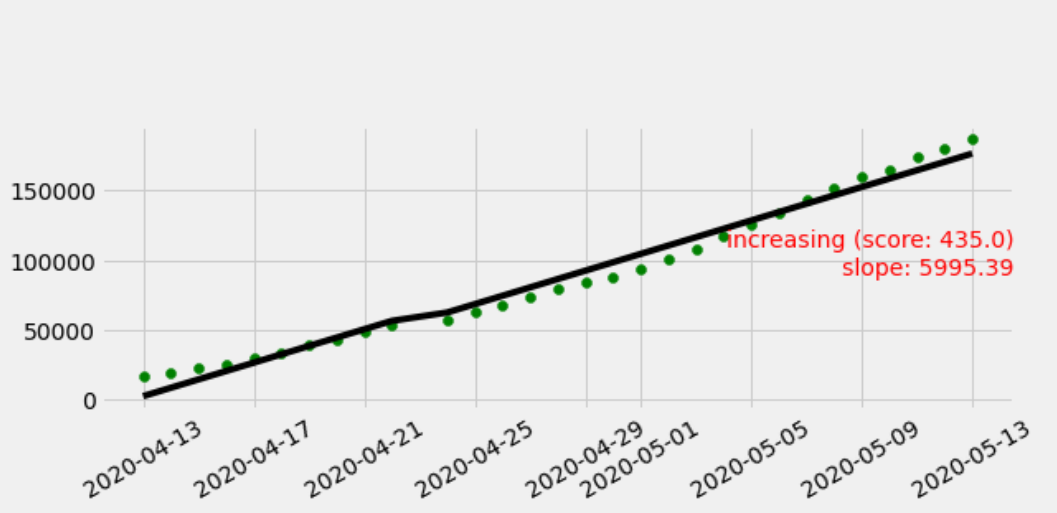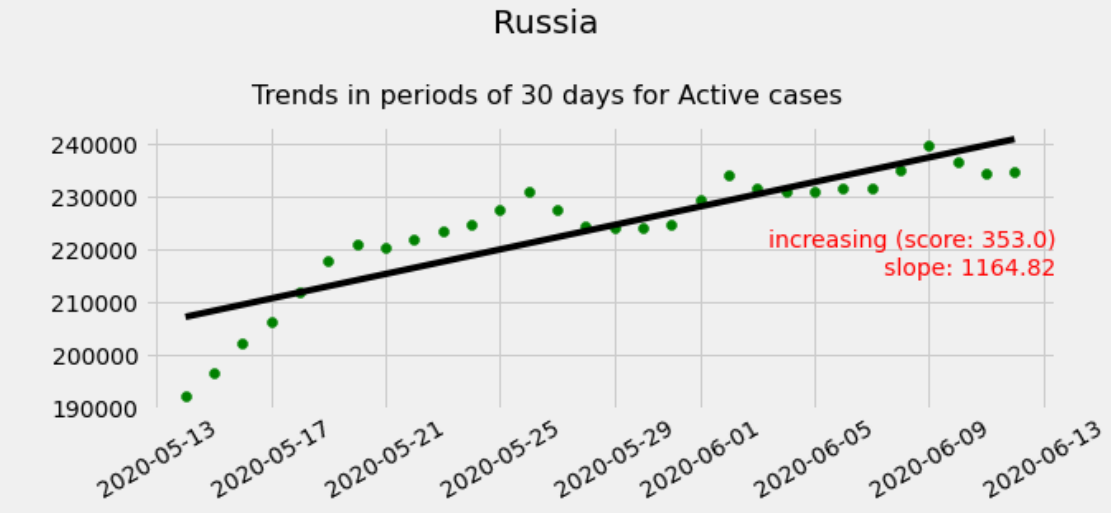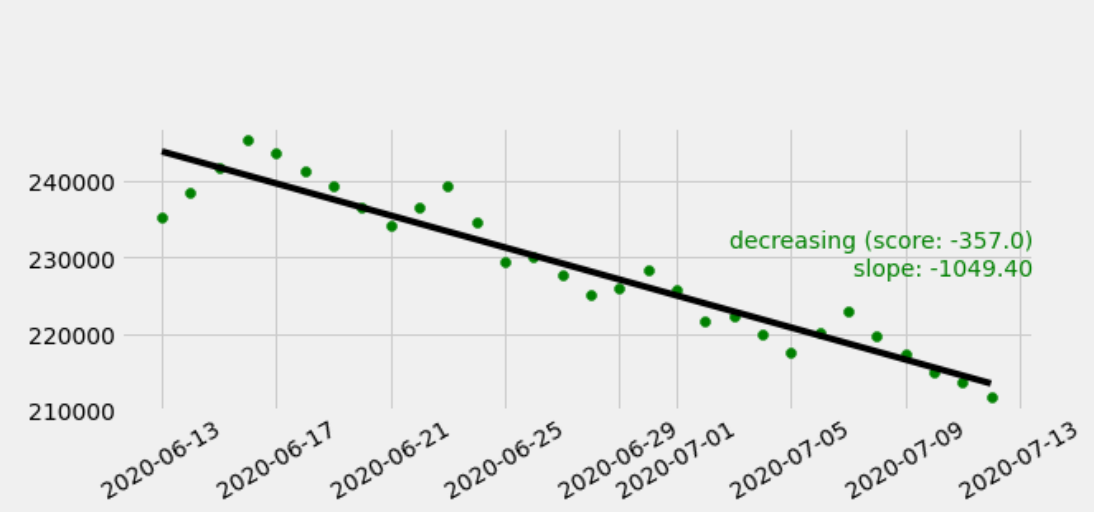OK, if you are interested in how we generated the above diagrams, just check this notebook on Google Colab. There you can find similar diagrams for all countries in the world depicting the monthly trend of active Covid-19 cases.

You can generate in our Google Colab notebook with a single line of code a trend image with a command like this one:

TrendDrawer('Russia', 'Active', 30, diagram_count=6).draw()

After running our notebook, we counted the type of trend per country and got this result:

 Trend Type Number of Countries increasing 122 decreasing 54 no trend 42 not enough data 19

### Conclusion

If you want to create your own visualisations of data for Covid-19 you can do it by grabbing some publicly available data via git. Then by using a simple Jupyter notebook, you can analyse the trends by yourself. We used in our example ridge regression with scikit learn for drawing lines and the library pymannkendall for the Mann-Kendall Test. This trend test can be used for other problems as it works well for all distributions. It also exists in different variations (also variations that target seasonability bound distributions), but we just used the basic version of it in our exercise.